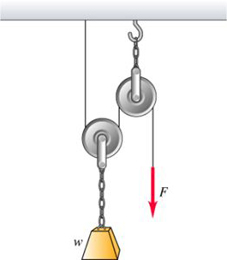Question# In the figure a worker lifts a weight \omega by pulling down on a rope with a force \vec{F}. The upper pulley is attached to the ceiling by a chain,an

Other
ANSWEREDIn the figure a worker lifts a weight $$\displaystyle\omega$$ by pulling down on a rope with a force $$\displaystyle\vec{{{F}}}$$. The upper pulley is attached to the ceiling by a chain,and the lower pulley is attached to the weight by another chain.The weight is lifted at constant speed. Assume that the rope,pulleys, and chains all have negligible weights.A) In terms of $$\displaystyle\omega$$,find the tension in the lower chain.
B) In terms of $$\displaystyle\omega$$,find the tension in upper chain.
C) In terms of $$\displaystyle\omega$$,find the magnitude of the force $$\displaystyle\vec{{{F}}}$$ if the weight is lifted at constant speed.2021-05-10
Newton’s 3rd law states that for every action, there is always an equal and opposite reaction. Thus, on the lower chain, the force action on it is w in the downward direction.
So, according to the Newton’s 3rd law, there must be an equal force in the opposite direction. The opposite force balancing the downward force is called as tension in the lower chain.
It is mathematically given by,
$$\displaystyle\vec{{{F}}}=\vec{{{T}}}$$
Substitute w for F, where w is the weight which acts as force. T is tension.
$$\displaystyle\omega=\vec{{{T}}}$$
Thus, the tension in the lower chain is w.
Part A:
According to the Newton’s 3rd law, force in the downward direction is always balanced equally by the force in the upward direction. Here, the force in the upward direction is in the form of tension. So, the tension in the lower chain is w.
The diagram given above shows the individual forces acting on the upper chain. Here, the force acting on the upper chain is the result of the half force applied by the person and half by the lower chain.
Thus, the force applied by the upper chain will be half of the original one. Thus, $$\displaystyle{\frac{{\omega}}{{{2}}}}$$.
Similarly, the force applied by the lower chain is given by, $$\displaystyle{\frac{{\omega}}{{{2}}}}$$.
Thus, the total force on the upper chain is given by,$$\displaystyle{\frac{{\omega}}{{{2}}}}+{\frac{{\omega}}{{{2}}}}=\omega$$
So, force on the upper chain is w.
Part: B
The force on the upper chain is because of the lower chain as well as the force applied by the person. So, the contribution from both the factors is half-half.
In the upper pulley, you do not gain any mechanical advantages, however, the lower pulley reduces the required force by 1/2.
Thus, the magnitude of the force $$\displaystyle\vec{{{F}}}$$ if the weight is lifted at constant speed will be $$\displaystyle{\frac{{{1}}}{{{2}}}}\omega$$
Part C:
The magnitude of the force if the weight is lifted at constant speed will always be the half. It is seen from the diagram given above that the individual force acting on the spring is half, as other half of the force will be balanced by the opposing force from the chain.